×#### Thank you for registering.

One of our academic counsellors will contact you within 1 working day.

Click to Chat

1800-1023-196

+91-120-4616500

CART 0

• 0

MY CART (5)

Use Coupon: CART20 and get 20% off on all online Study Material

ITEM
DETAILS
MRP
DISCOUNT
FINAL PRICE
Total Price: Rs.

There are no items in this cart.
Continue Shopping• Complete JEE Main/Advanced Course and Test Series
• OFFERED PRICE: Rs. 15,900
• View Details

Revision Notes on Complex Numbers

z1 = a + ib and z2 = c + id then z1 = z2 implies that a = c and b = d.i2 = –1, i3 = – i, i4 = 1, i4n+1 = i, i4n+2 = – 1, i4n+3 = – i, i4n = 1

z1 + z2 = (Re(z1) + Re (z2)) + i (Im (z1) + Im (z2))

z1 – z2 = (Re(z1) – Re(z2)) + i (Im (z1) - Im (z2))

Re (z1. z2) = Re (z1). Re(z2) – Im(z1). Im (z2)

Im (z1. z2) = Im(z1). Re(z2) + Im(z2). Re(z1)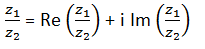Square root of a complex number: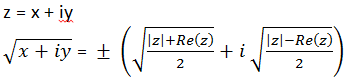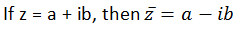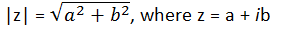Argument of a Complex Number:

1. Argument of a complex number p(z) is defined by the angle which OP makes with the positive direction of x-axis.

2. Argument of z generally refers to the principal argument of z (i.e. the argument lying in (–π, π) unless the context requires otherwise.

General argument: General value of argument = 2nπ + θ; where θ is one of the argument of z.

Amplitude (Principal value of argument):

z = a + ib, then calculate ∝ = tan-1 |b/a|.

Then consider the following cases:

amp (z) = θ  = ∝

amp (z) = θ = (π – ∝)

amp (z) = θ = – (π – ∝)

amp (z) = θ = – ∝

?Least  Positive argument: Value of θ such that 0 < θ ≤ 2π

If OP = |z| and arg (z) = θ, then obviously z = r (cos θ + i sin θ) and is called the polar form of complex number z.

|(z-z1) / (z-z2)| =1 the locus of point representing z is the perpendicular bisector of line joining z1 and z2.

-|z| ≤ Re(z) ≤ |z| and -|z| ≤ Im(z) ≤ |z|

If a and b are real numbers and z1 and z2 are complex numbers then |az1 + bz2|2 + |bz1 - az2|2 = (a2 + b2) (|z1|2 + (|z2|2)

The distance between the complex numbers z1 and z2 is given by |z1 - z2|.

In parametric form, the equation of line joining z1 and z2 is given by z = tz1 + (1 – t)z2.

If A(z1) and B(z2) are two points in the argand plane, then the complex slope μ of the straight line AB is given by μ, where μ =Two lines having complex slopes μ1 and μ2 are:

1. Parallel iff μ1 = μ2

2. Perpendicular iff μ1 = - μ2 or μ1 + μ2 = 0

If A(z1), B(z2), C(z3) and D(z4) are four points in the argand plane, then the angle θ between the lines AB and CD is given by θ = arg{(z1 - z2)/ (z3 – z4)}

Some basic properties of complex numbers:

I. ||z1| - |z2|| = |z1 + z2| and |z1 - z2| = |z1| + |z2| iff origin, z1, and z2 are collinear and origin lies between z1 and z2.

II. |z1 + z2| = |z1| + |z2| and ||z1| - |z2|| = |z1 - z2| iff origin, z1 and z2 are collinear and z1 and z2 lie on the same side of origin.

III.amp (z1 z2) = amp (z1) + amp (z2) + 2kπ, k ∈ I

IV. amp(zn) = n amp z

V. The least value of |z - a| + |z - b|  is |a - b|.Demoivre's Theorem: The theorem can be stated in two forms:

Case I: If n is any integer, then

(i) (cos θ + i sin θ)n = cos nθ + i sin nθ

(ii) (cos θ1 + i sin θ1) . (cos θ2 + i sin θ2) ......... (cos θn + i sin θn) = cos (θ1 + θ2 + θ3 .................. + θn) + i sin (θ1 + θ2 + ..............  θn)

Case II: For p and q such that q ≠ 0, we have

(cos θ + i sin θ)p/q = cos((2kπ + pθ)/q) + i sin((2kπ + pθ/q)  where k = 0,1,2,3,....., q-1

Demoivre’s formula does not hold for non-integer powers.

Main application of Demoivre’s formula is in finding the nth roots of unity. So, if we write the complex number z in the polar form then,

z = r(cos x + isin x)

Then z1/n = [r (cos x + i sin x)]1/n

= r 1/n [ cos (x + 2kπ/n) + i sin (x + 2kπ/n)]

Here k is an integer. To get the n different roots of z one only needs to consider values of k from 0 to n – 1.

Cube roots of unity

1, ω, ω 2 are the 3 cube roots of unity

1 + ω + ω 2 = 0 & ω 3 = 1

1 + ω r + ω 2r = 0 if r is not multiplier of 3

or 3 if r is multiple of 3

n, nth roots of unity 1, α, α² . . . . αn are n nth roots of unity, then 1 + α + α² + . . . . . + αn – 1 = 0.

Continued product of the roots of a complex quantity should be determined using theory of equations.

The only complex number with modulus zero is the number (0, 0).

The following figures illustrate geometrically the meaning of addition and subtraction of complex numbers:Different forms of equation of circle:

(i) |z| = r, r ∈ R+ then locus of z represent a circle whose centre is the origin and radius is equal to r.

(ii) |z – z0| = r, r ∈ R+ then locus of z represents a circle whose centre is z0 and radius is equal to r.

(iii) Equation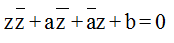represents a circle whose centre is z0 and radius is equal to r.

The formulae for centroid, incentre and orthocentre are as given below: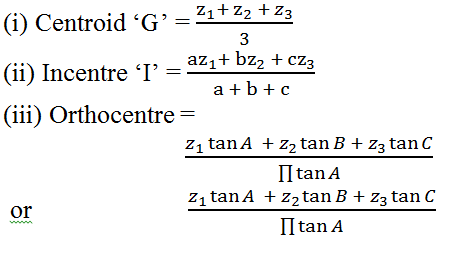### Course Features

• 728 Video Lectures
• Revision Notes
• Previous Year Papers
• Mind Map
• Study Planner
• NCERT Solutions
• Discussion Forum
• Test paper with Video Solution